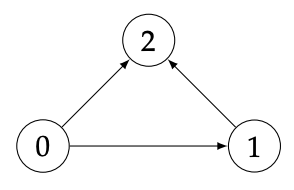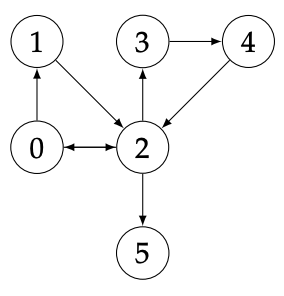시간 제한 메모리 제한 제출 정답 맞은 사람 정답 비율
3 초 512 MB 71 28 28 50.909%

## 문제

Kiara studies an odd species of birds which travel in a very peculiar way. Their movements are best explained using the language of graphs: there exists a directed graph G where the nodes are trees, and a bird can only fly from a tree Ta to Tb when (Ta, Tb) is an edge of G.

Kiara does not know the real graph G governing the flight of these birds but, in her previous field study, Kiara has collected data from the journey of many birds. Using this, she has devised a graph P explaining how they move. Kiara has spent so much time watching them that she is confident that if a bird can fly directly from a to b, then she has witnessed at least one such occurrence. However, it is possible that a bird flew from a to b to c but she only witnessed the stops a and c and then added (a, c) to P. It is also possible that a bird flew from a to b to c to d and she only witnessed a and d, and added (a, d) to P, etc. To sum up, she knows that P contains all the edges of G and that P might contain some other edges (a, b) for which there is a path from a to b in G (note that P might not contain all such edges).

For her next field study, Kiara has decided to install her base next to a given tree T. To be warned of the arrival of birds on T, she would also like to install detectors on the trees where the birds can come from (i.e. the trees T' such that there is an edge (T', T) in G). As detectors are not cheap, she only wants to install detectors on the trees T' for which she is sure that (T', T) belongs to G.

Kiara is sure that an edge (a, b) belongs to G when (a, b) is an edge of P and all the paths in P starting from a and ending in b use the edge (a, b). Kiara asks you to compute the set S(T) of trees T' for which she is sure that (T', T) is an edge of G.

## 입력

The input describes the graph P. The first line contains three space-separated integers N, M, and T: N is the number of nodes of P, M is the number of edges of P and T is the node corresponding to the tree on which Kiara will install her base.

The next M lines describe the edges of the graph P. Each contains two space-separated integers a and b (0 ≤ a, b < N and a ≠ b) stating that (a, b) ∈ P. It is guaranteed that the same pair (a, b) will not appear twice.

## 출력

Your output should describe the set S(T). The first line should contain an integer L, which is the number of nodes in S(T), followed by L lines, each containing a different element of S(T). The elements of S(T) should be printed in increasing order, with one element per line.

## 제한

• 1 ≤ N, M ≤ 100 000;
• 0 ≤ T < N.

## 예제 입력 1

3 3 2
0 1
0 2
1 2


## 예제 출력 1

1
1The graph corresponding to this example is depicted on the right. The node 1 belongs to S(2) because the (only) path from 1 to 2 uses (1, 2). The node 0 does not belong to S(2) because the path 0 → 1 → 2 does not use the edge (0, 2).

## 예제 입력 2

6 8 2
0 1
0 2
1 2
2 0
2 3
3 4
4 2
2 5


## 예제 출력 2

2
1
4The graph corresponding to this example is depicted on the right. For the same reason as in Sample 1, the node 0 does not belong to S(2) while 1 does. The nodes 3 and 5 do not belong to S(2) because we do not have edges (3, 2) or (5, 2). Finally 4 belongs to S(2) because all paths from 4 to 2 use the edge (4, 2).